# 6.1: Surge Tank Model

•• Contributed by Peter Woolf et al.
• Assistant Professor (Chemical Engineering) at University of Michigan

Authors: (September 6, 2007) Cara Canady, David Carpenter, Che Martinez, Jeremy Minty, Bradley Novak

## 1.1 Introduction

Used to regulate fluid levels in systems, surge tanks act as standpipe or storage reservoirs that store and supply excess fluid. In a system that has experienced a surge of fluid, surge tanks can modify fluctuations in flow rate, composition, temperature, or pressure. Typically, these tanks (or “surge drums”) are located downstream from closed aqueducts or feeders for water wheels. Depending upon its placement, a surge tank can reduce the pressure and volume of liquid, thereby reducing velocity. Therefore, a surge tank acts as a level and pressure control within the entire system.

Since the flow to the surge tank is unregulated and the fluid that is output from the surge tank is pumped out, the system can be labeled as unsteady-state [MIT], but the approach to an approximate solution (below) utilizes techniques commonly adhered to when solving similar steady-state problems.

The technology behind surge tanks has been used for decades, but researchers have had difficulty fully finding a solution due to the non-linear nature of the governing equations. Early approximations involving surge tanks used graphical and arithmetical means to propose a solution, but with the evolution of computerized solving techniques, complete solutions can be obtained. [Wiley InterScience].

## 1.2 Derivation of Ordinary Differential Equation

To accurately model a surge tank, mass and energy balances need to be considered across the tank. From these balances, we will be able to develop relationships for various characteristics of the surge tank

### 1.2.1 Diagram of Surge Tank SystemA surge tank relies on the level sensor to determine whether or not fluid stored in the tank should be removed. This regulated outflow is pumped out in response to calculations made by a controller that ultimately opens and closes the control valve that releases the fluid from the tank.

### 1.2.2 Governing Equations of Surge Tank Model

A surge tank's components must be divided up and evaluated individually at first, then as a whole. First, the expression for the inlet stream must be obtained. The simplistic sine function will be used as the basis for the expression of a stream because it typically describes the tidal surge pattern of a low-viscous fluid, like water. The flowrate, w, will be given in units of kg h-1.

$w_{i}=a+b \sin (c \pi t)$

where a and b are constants determined by the specific problem circumstance.

A mass balance must now be performed on the tank as a system. Using the concept that mass in = mass out, and assuming that the tank is a perfect cylinder devoid of diversions, an expression can be derived:

rate mass in - rate mass out = accumulated fluid in tank

Rewriting,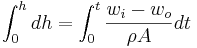Where, at time t=0, the amount of fluid in the surge tank is constant; thus h(0) = h0.

Substituting the original equation for the inlet stream, wi, into the expression for the height of the tank, h(t), the governing equation for the height of the tank is obtained:

$h(t)=h_{o}+\frac{1}{\rho A} \int_{0}^{t}\left[\left(a+b \sin (c \pi t)-w_{0}\right] d t\right.$

Integrating by parts,

$h(t)=h_{o}+\frac{x}{\rho A}(1-\cos (c \pi t))$

where x is formed from the constants a and b during integration.

## 1.3 Secondary uses of Surge Tanks

Surge tanks are most commonly used to protect systems from changes in fluid levels; they act as a reservoir that stores and supply excess fluid. In addition, the tanks shield the systems from dramatic changes in pressure, temperature, and concentration. They can also allow one unit to be shut down for maintenance without shutting down the entire plant.

### 1.3.1 Pressure

There can be moments of high pressure, called hammer shock, in a system when the liquid (incompressible) flow is stopped and started. The energy that liquids possess while traveling through pipes can be categorized as potential or kinetic. If the liquid is stopped quickly, the momentum that the liquid carries must be redirected elsewhere. As a result, the pipes vibrate from the reactive force and weight of the shock waves within the liquid. In extreme cases, pipes can burst, joints can develop leaks, and valves and meters can be damaged.

The extreme amounts of pressure are dampened when the fluid enters the surge tanks. The surge tank acts as a buffer to the system, dispersing the pressure across a greater area. These tanks make it possible for a system to more safely execute their tasks.

### 1.3.2 Temperature

The temperature of a fluid can either be controlled or changed through the use of a surge tank. The surge tank allows for a rapid change in fluid temperature. This is exemplified by the process of pasteurization; the milk needs to be at a high temperature for just a short period of time, so it is exposed to the high temperature and then moved to the surge tank where it can be stored and cooled (see heated surge tank).

A substance can enter the surge tank at room temperature, and it will instantaneously mix with the rest of the tank. Substances entering the tank will also subsequently rise to meet the high temperature and then exit the surge tank quickly thereafter.

### 1.3.3 Concentration

Concentration inside the surge tank is kept relatively constant, thus the fluid exiting the surge tank is the same as the fluid in the tank. This is favorable when there is a concentration gradient in the incoming fluid to the surge tank. The tank homogenizes the entering fluid, keeping the concentration of the reactants the same throughout the system, therefore eliminating any concentration gradient.

Example $$\PageIndex{1}$$: Modeling Surge Tank

Suppose we are to design a surge tank that deals with flow swings of +/- 40% over a 10 minute period modeled by the following equation:

$w_{1}=500+200 \sin \frac{\pi t}{10} \label{1}$

where flow is m3h-1 and time in hours. The outlet flow rate, wo, is desired to be 500 m3h-1.

The surge tank is 30m tall and has a cross sectional area of 5m2. The initial height of the fluid (ρ = kg / m3) is 10m.

1. Model this hypothetical example.
2. The surge tank has upper and lower limit controls that open or close the valve if the level gets too high or low. What is the highest maximum set point that can be used without the surge tank overflowing?

Solution

[A.] First we should write out the Material Balance:

$\rho A \frac{d h}{d t}=w_{i}-w_{o} \label{2}$

with $$h(0)=h_{o}$$.

$h(t)=h_{o}+\frac{1}{\rho A} \int_{0}^{t}\left(w_{i}(t)-w_{o}(t)\right) d t$

Substituting Equation \ref{1} into \ref{2}, we get

$h(t)=h_{o}+\frac{2000}{\rho \pi A}\left(1-\cos \frac{2 \pi t}{20}\right) \label{3}$

To complete the design, we must have the cross-sectional area A of the surge tank, this would be given.

If we were to apply the following condition,

$w_{i}-w_{o}=C \label{4}$

and were to substitute Equation \ref{4} into Equation \ref{2}, we discover

$h(t)=h_{o}+\frac{C}{\rho A} t \label{5}$

[B.] Using the Microsoft Excel model provided, the highest possible maximum set point is 23m. Media:Model Surge Tank.xlsExample $$\PageIndex{2}$$

In this example, we simulate random fluctuations that might be observed in a real world process stream. A random number generating function (in MS Excel) is used to generate pseudo-random fluctuations of200 kg/h about an average value of 1000 kg/h for the inlet stream, wi(t) over a period of 5 hours. The result is as follows:Examine the effect that these fluctuations have on the fluid level in surge tanks of various volumes. To vary the volume, assume tanks of constant height hmax=20m, and vary the cross sectional area from A=1m2 to A=5m2. Use the following parameters for the surge tank: Initial fluid level h0=10m, ρ=1 kg / m3, w0=1000 kg / m3, t0=0 h and tf=5h.

Solution

In the solution to the first example problem, we used a trigonometric function to simulate fluctuations in the inlet stream, and we obtained an analytical solution to the differential equation. Since we are using a pseudo-random fluctuating inlet stream in this example, we will solve this problem via numerical methods. Using the pseudo-random data for wi(t), we perform a numerical integration on the previously derived expression:

$h(t)=h_{o}+\frac{1}{\rho A} \int_{0}^{t}\left(w_{i}(t)-w_{o}(t)\right) d t \labl{ex2:2}$

This integration was performed with the trapezoid rule (in MS Excel, using a slight modification of the posted Excel model), using the specified surge tank parameters, with A=1 m2, 2 m2, and 5 m2 l. The following results were obtained: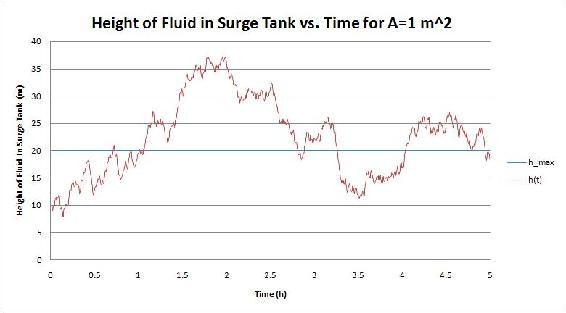We see that increasing the volume of the surge tank by increasing the cross sectional area A reduces the magnitude of the fluctuations of the fluid level h in the surge tank. For A=1 m2 and 2m2, the capacity of the surge tank is exceeded and it overflows.

Example $$\PageIndex{3}$$

An operator quickly adds 50 gallons from a drum of water into a cylindrical surge tank with a diameter of 4 feet. The initial volume of liquid in the tank is 40 cubic feet and the total height of the tank is 5 feet. The inflow and exit flow rates are initally 6 cubic feet per minute. The resistance has a linear relationship with liquid height.

1. Derive a first order linear model for the tank height and determine the value of R for the outflow
2. Will the tank overflow when the drum is added?
3. Show the height h(t) after the drum of water is added; treat the change in h(t) as instantaneous

Solution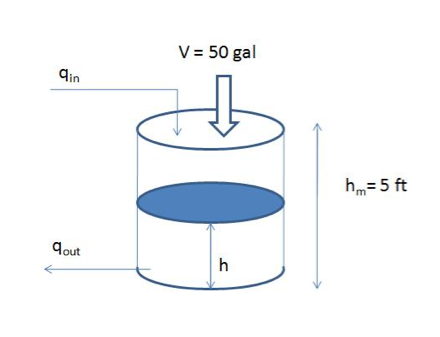(a)

Assume the operator adds the water at t = 0, so when t < 0, the tank is under steady state which can be described as:qINqOUT = 0 (1)

Assume:where R is the resistance and h is the height of the liquid. Initial volume of the tank is: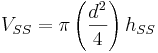Thus:Substituteand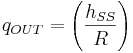into equation 1 above:

0 = 6 − hSS / RAt time t > 0, the 50 gallons (6.685 cubic feet) of water is added to the system which disrupts the steady state and the corresponding initial condition of the system is:

V0 = VSS + Voperator = 40 + 6.685 = 46.685ft3The corresponding dynamic linear model:

$A\left(\frac{d h}{d t}\right)=q_{I N}-q_{O U T}=q_{I N}-\left(\frac{h}{R}\right) \label{ex2:2}$

with initial condition: $$h_0 = 3.7151\,ft$$

(b)

After adding the drum, h0 = 3.7151 which is lower than 5 feet. The system will not overflow at t0. Because the liquid level is higher than the steady state level and, the out flow rate will be larger than qIN which pushes the liquid level drop. As a result, water will not over flow.

(c)

Define deviation variables. h' = hhSS ; qIN' = qINqINSS = 0 and substitute into equation (2)(3)

Solve the linear model above (Eq. 3): (Either by direct integration or laplace transform and inverse lapace transform)(4)

Put h' = hhSS into equation 4:Then:(d)

Yes. Since

$\lim _{t \rightarrow \infty} h=3.183+0.5321 \exp (-0.1499 t)=3.183 \mathrm{ft}$

which was the original steady state